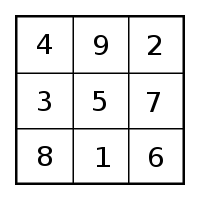# Fibonacci Hidden Content in 1st 2 Number Squares

Fibonacci Hidden Content in Number Squares. Number squares revive a lost link with the past. A number of these blogs demonstrate their use in antiquity. First, what is the Fibonacci series of numbers?

### Beethoven: His Fibonacci Fifth – DSO Works

In mathematics, the Fibonacci numbers are in the following integer sequence.   Every number after the first two is the sum of the two preceding ones: 1,\;1,\;2,\;3,\;5,\;8,\;13,\;21,\;34,\;55,\;89,\;144/;233/; 377…

The ratio of the 2nd to the 1st initiates a spiral of natural growth. Craftsmen across many disciplines use the Fibonacci series. Beethoven is included in the list. As the numbers grow the successive addition, the second divided by the first gets closer and closer to the “Golden Section”. That ratio is 1 to 1.618….

Beethoven: His Fibonacci Fifth – DSO Works
Do musicians really work with the “life force?”
How Fibonacci hidden content are Found in the 1st two Number Squares

Most significant, the 3 x 3 number square sets a framework of fives. This is accomplished by hidden number codes. The 4 x 4 number square fills the fives in with consecutive numbers. Here’s how:

### How Fibonacci hidden content are Found in the 1st two Number Squares13, 21 and 34 are key numbers on this square- all three are FibonacciThese 1st two number squares, 3 x 3 and 4 x 4, set the entire pattern of the  cosmos. Ancients knew this. We don’t.

### Solving the Mystery of Hidden Fibonacci Content

“5” is the core of the 3 x 3 number square. In the sequence above, it is the 1st non consecutive number. We have 1,1,2,3,5,8, etc. 55 is another hidden Fibonacci number. It requires a simple mathematical operation. Take 95 + 15. That equals 110. Divide that by two. That equals 55. That 110 total can be found is 15 more ways on this number square. See how many you can find. Be stubborn. Keep in mind 16 x 110 = 1,760. That’s the number of yards in a mile. That’s also the number of shorter Egyptian cubits around the Great Pyramid.

On the 4 x 4 square of numbers note: 1 + 12 =13. Adjacent we have 15 +6 = 21.  Any row of four number totals 34. These are all Fibonacci numbers. More to follow in future posts.

Jupiterian Hidden Harmony in a Number Square